## A NOTE ON NOTATION

Introduction

THE basic notation of this book will be the Łukasiewicz parenthesis-free notation. Many logicians, unfortunately, are familiar with this 'Polish notation' only in so far as they know of its existence; these people might find it difficult to believe that the Łukasiewicz notation is, with a little practice, at least as easy to read and use as is the 'standard' notation, particularly for work with propositional calculi and modal systems; this is reflected in current literature in this area; a very large amount of research in systems of the kind investigated in this book is published in the Polish notation-in which, then, the would-be student of such systems must be fluent.

The researcher himself gains an obvious advantage in his choice of this notation, for almost all of the characters he will use in setting down his results are to be found on a standard typewriter keyboard. But the notation gives him another more important advantage. It lends itself exceptionally well to the use of the rules of inference commonly used with propositional calculi : substitution for variables and detachment.

Detachment, substitution, and 'application'

The rule of substitution is, of course, the permission to replace all occurrences of a given variable (p, q, r, . . . ) in a given formula (specifically, a formula which is a step in a deduction of some sort) by any well-formed formula (abbreviated 'wff') of the system. Detachment, sometimes called modus ponens, is the permission to move from the assertion of the formula j and that of the formula read as 'if j then y' to the assertion of y. The formula read as 'if j then y' is, of course, j É y in the 'standard' notation; in our notation it will be Cjy. 'C', typical of Łukasiewicz binary operators, is to be thought of as being followed by two blanks, into each of which is inserted a wff of the system. In the case of 'C', the 'sign of implication', the leftmost of these formulas is called the 'antecedent' and the other the 'consequent' of the whole implication.

To use the rule of detachment, we must find an asserted formula j and another asserted formula of form Cjy. Most commonly, finding such formulas involves the use of the rule of substitution. We shall usually have to work initially with two asserted formulas -- say, j and Ccy -- with c, in the majority of cases, not the same formula as j. When j and c are not the same, we must find substitutions into one or both of j and Ccy which will change j and c into the same formula. Consider a simple case: let Ccy and j be the formulas

 1 CCpCqrCCpqCpr 2 CpCqp

respectively. We note first that since the principal connective of a Polish-notation formula is always its first character, the antecedent of 1 -- as of every implication -- begins with the second character of 1. We then begin matching 1's characters with those of 2, starting with the second character of 1 and the first of 2, thus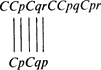When the matching has exhausted all the characters of 2, the next unused character of 1 is the first character of 1's consequent. The matching procedure obviously tells us here that 2 and the antecedent of 1 may be made identical by substituting p for r in formula 1. We may then write the result of the substitution and the following detachment simply by writing out the consequent of 1, making as we do the required substitution

 3 CCpqCpp

We may carry the process a little further by now allowing formula 3 to be our Ccy and formula 2 again to be our j. Again we match the antecedent -- of 3 this time -- with formula 2: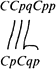Here we encounter a slightly different situation: the q in 3 matches not a variable in 2 but a C. In this case we shall consider that the variable q is correlated not with the C alone, but with the whole wff of which the C is the first and main connective. Obviously again, the matching procedure tells us that the antecedent of 3 may be made the same formula as 2 simply by substitution of Cpq for q in 3. Since q does not occur in 3's consequent, the result of so substituting and then detaching is the formula

 4 Cpp

It is simplest, I think, to consider the making of requisite substitutions and the subsequent detachment as in the above two examples to be one operation. We may call this operation 'application', and we shall say that 3 was the result of the application of 1 to 2, and that we get 4 by applying 3 to 2. We apply one asserted formula j to another y, then, by finding substitutions in one or both formulas which will turn the antecedent of j into the same form as y and -- once we have made the substitution -- by making use of the rule of detachment. Let us consider another example; take the formula

 5 CCpqCCqrCpr

and apply it to itself:Let us use the notation 'p/j' to mean that we substitute j for the variable p; in one instance of 5 (that instance which plays the role of the earlier-discussed Ccy) then, we must make the substitutions p/Cpq and q/CCqrCpr. We may then write the result of the application of 5 to itself as follows

CCCCqrCprrCCpqr.

But wait -- the above formula is not exactly what we want. Suppose that before we had applied the first instance of 5 to the second, we made the substitution r/s in the first instance, and then went on with the application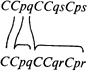The result of the application would then be

 6 CCCCqrCprsCCpqs

Note that 6 contains four distinct variables, while our first attempt to get 6 contains only three. 6 is a more general formula than is CCCCqrCprrCCpqr; this is easily seen by noting that we can always, by s/r, get from 6 to this latter formula-but not vice versa. We shall, in fact, want so to manage our applications of formulas to other formulas that we shall always get the most general results of these applications, that is, the formulas with the largest possible numbers of distinct variables. How do we guarantee this? The answer is simple. When we apply a formula j to another formula y, we shall -- before matching j's antecedent with y -- make substitutions of variables for variables into one of the formulas so that the two formulas will have no variables in common. In practice this is easily done by attaching an index of some sort to each of the variables in, say, j and then applying the resulting formula to y. A simple method of doing this is to place a dot over each of j's variables; in the case of 5's application to itself, this gives us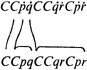Our substitutions are now ˙p / Cpq and ˙q / CCqrCpr. The result of the application is then

CCCCqrCpr˙rCCqp˙r;

in this formula ˙r is immediately recognized as distinct from r; for ease in reading and writing, we now make the substitution ˙r / s, giving us 6.

The 'D-notation'

We are now in a position to describe a means of very compactly justifying lines in a deduction (see Prior, 1962). Let x and y be the numbers of two formulas occurring in a deduction; we shall then write

Dxy = z

to mean that z is the number of the most general result of applying the formula x to the formula y. Thus, formula 4 above is D32.

We may think of D as a function which takes formula numbers into formula numbers; since with our above numbering D12 = 3, we might represent the entire deduction of formula 4 very compactly by DD122 = 4. We shall make considerable use of the D-notation and variants thereof in our proof justification. (Of course, we shall make use of other methods of justification; often, for example, we shall justify a proof line simply by referring to one or more previous lines and letting the reader himself supply the specific means of movement to the line in question.)

Now let us do something at first glance unsightly and seemingly devoid of promise; we perform the deduction D66: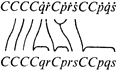The procedure for this more complex application is the same as for the simpler ones -- but here some new points are illustrated. New here is the fact that a variable in the lower instance, s, matches a larger formula, C˙p˙r , in the upper instance. This means, of course, that here substitutions must be made into the lower instance of 6 as well as the upper (inexperienced students are tempted to say, 'I shall substitute s for C˙p˙r  in the upper instance'. This is a terrible mistake; the only thing that the rule of substitution permits you to substitute for is variables). The indicated substitutions are, in the upper instance

 (a) ˙q / Cqr (b) ˙r / Cpr (c) ˙s / CCpqs

and in the lower

 (d) s / C˙p˙r

But we are not yet finished. Note that in substitution (c) an s occurs, while s itself is the subject of substitution (d); in (d) itself, ˙r occurs, while ˙r is the subject of substitution (b). Since the s in (c) is the same as that substituted for in (d), it must have the same formula substituted for it as does s in (d). This would be the formula C˙p˙r; here, however, be careful. The ˙r in (d) is the same as that in (b); thus, it too must be substituted for. We correct (d) first

(d') s / C˙pCpr ;

we may now write the final version of (c), replacing its s as indicated in (d):

(c') ˙ / CCpqC˙pCpr.

We are now prepared to write out the formula D66; remember, all we need do is reproduce the consequent of the upper instance of 6 with the indicated substitutions (the reader may verify for himself that the lower instance of 6 and the antecedent of the upper are the same formula given substitutions (a), (b), (c'), and (d'))

CC˙pCqrCCpqC˙pCpr;

for convenience in reading, we reletter this formula with ˙p / pp / s:

 7 CCpCqrCCsqCpCsr

Formula 7 is much more civilized in appearance than is 6 and is, in fact, deductively 'loaded'; a number of important theorems of the propositional calculus are 'hiding' in 7, waiting to be made explicit with just a little manipulation.

This manipulation, however, is not our immediate purpose. What we wanted to do was to get across something of the feel of the Łukasiewicz notation. The only real way to gain familiarity with any notation is to use it; this is also the only way of finding the ins and outs of applying formulas to each other. One should not be surprised at finding out that there are formulas which simply do not apply to each other, for example; if a person works even a little while at finding proofs, he will discover an ample number of these and of other problems of the deducer. To become master of all these problems requires far more than one can expect to have absorbed from these few pages -- but the reader should certainly be able, now, to follow with some facility the arguments and proofs that follow.